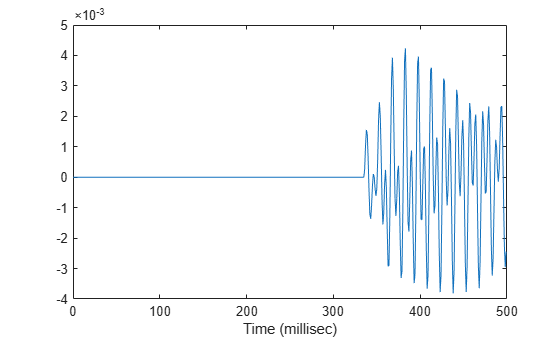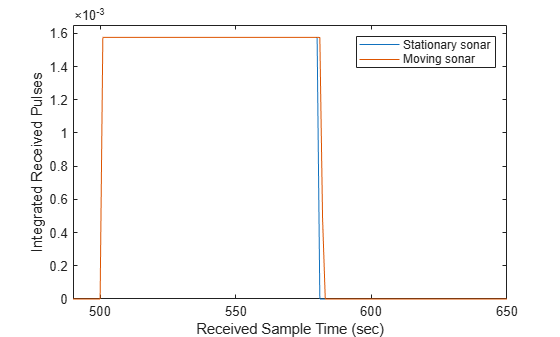# phased.IsoSpeedUnderwaterPaths

Isospeed multipath sonar channel

## Description

The `phased.IsoSpeedUnderwaterPaths` System object™ creates an underwater acoustic channel to propagate narrowband sound from point to point. The channel has finite constant depth with air-water and water-bottom interfaces. Both interfaces are planar and horizontal. Sound speed is constant throughout the channel. The object generates multiple propagation paths in the channel using the acoustical method of images (see ). Because sound speed is constant, all propagation paths are straight lines between the source, boundaries, and receiver. There is always one direct line-of-sight path. For each propagation path, the object outputs range-dependent time delay, gain, Doppler factor, reflection loss, and spreading loss. You can use the channel data as input to the multipath sound propagator, `phased.MultipathChannel`.

To model an isospeed channel :

1. Define and set up the channel. You can set `phased.IsoSpeedUnderwaterPaths` System object properties at construction time or leave them to their default values. See Construction. Some properties that you set at construction time can be changed later. These properties are tunable.

2. To create the multipath channel, call the `step` method of `phased.IsoSpeedUnderwaterPaths`. The output of the method depends on the properties of the `phased.IsoSpeedUnderwaterPaths` System object. You can change tunable properties at any time.

Note

Instead of using the `step` method to perform the operation defined by the System object, you can call the object with arguments, as if it were a function. For example, `y = step(obj,x)` and `y = obj(x)` perform equivalent operations.

## Construction

`channel = phased.IsoSpeedUnderwaterPaths` creates an isospeed multipath underwater channel System object, `channel`.

`channel = phased.IsoSpeedUnderwaterPaths(Name,Value)` creates an isospeed multipath underwater channel System object, `channel`, with each specified property `Name` set to the specified `Value`. You can specify additional name and value pair arguments in any order as (`Name1,Value1`,...,`NameN,ValueN`).

## Properties

expand all

Channel depth, specified as a positive scalar. Units are in meters.

Example: `250.0`

Data Types: `double`

Underwater sound propagation speed, specified as a positive scalar. Units are in meter per second. The default value is a commonly-used underwater sound propagation speed.

Example: `1502.0`

Data Types: `double`

The source of the number of propagation paths, specified as `'Auto'` or `'Property'`. If you set this property to `'Auto'`, the object automatically determines the number of paths based on spreading and reflection losses. If you set this property to `'Property'`, you specify the number of paths using the `NumPaths` property.

When `NumPathsSource` is set to `'Auto'`, only paths having a total loss greater than 20 dB below the direct path loss are returned.

Example: `'Property'`

Data Types: `char`

The number of propagation paths, specified as a positive integer between 1 and 51, inclusive.

Example: `11`

#### Dependencies

To enable this property, set the `NumPathsSource` property to `'Property'`.

Data Types: `double`

Channel coherence time, specified as a nonnegative scalar. Coherence time is a measure of the temporal stability of the channel. The object keeps a record of cumulative step time. When the cumulative step time exceeds the coherence time, propagation paths are recomputed and the cumulative step time is reset to zero. If you set this quantity to zero, the propagation paths are update at each call to `step`. Units are in seconds.

Example: `5.0`

Data Types: `double`

Bottom reflection loss, specified as a nonnegative scalar. This value applies to each bottom reflection of a path. Units are in dB.

Example: `10`

Data Types: `double`

Frequencies for which to compute absorption loss, specified as a positive real-valued vector. Units are in Hz.

Example: `[1000:100:3000]`

Data Types: `double`

Enable two-way propagation, specified as a `false` or `true`. Set this property to `true` to perform round-trip propagation between the signal origin and destination specified in `step`. Set this property to `false` to perform only one-way propagation from the origin to the destination.

Example: `true`

Data Types: `logical`

## Methods

 reset Reset state of System object step Create propagation paths in an isospeed multipath sound channel
Common to All System Objects
`release`

Allow System object property value changes

## Examples

collapse all

Create a 5-path underwater sound channel and display the propagation path matrix. Assume the source is stationary and the receiver is moving along the x-axis towards the source at 20 kph. Assume one-way propagation.

```speed = -20*1000/3600; numpaths = 5; channelpaths = phased.IsoSpeedUnderwaterPaths('ChannelDepth',200,'BottomLoss',10, ... 'NumPathsSource','Property','NumPaths',numpaths,'CoherenceTime',5); tstep = 1; srcpos = [0;0;-160]; rcvpos = [500;0;-50]; srcvel = [0;0;0]; rcvvel = [speed;0;0]; pathmat = channelpaths(srcpos,rcvpos,srcvel,rcvvel,tstep); disp(pathmat)```
``` 0.3356 0.3556 0.4687 0.3507 0.3791 1.0000 -1.0000 -0.3162 0.3162 -0.3162 54.1847 54.6850 57.0766 54.5652 55.2388 ```

The first row contains the time delay in seconds. The second row contains the bottom reflection loss coefficients, and the third row contains the spreading loss in dB. The reflection loss coefficient for the first path is 1.0 because the direct path has no boundary reflections. The reflection loss coefficient for the second path is -1.0 because the path has only a surface reflection.

Create a 7-path underwater sound channel and display the propagation path matrix. Assume the source is stationary and the target is moving along the x-axis towards the source at 20 kph. Assume two-way propagation.

```speed = -20*1000/3600; numpaths = 7; channelpaths = phased.IsoSpeedUnderwaterPaths('ChannelDepth',200,'BottomLoss',10, ... 'NumPathsSource','Property','NumPaths',numpaths,'CoherenceTime',5,... 'TwoWayPropagation',true); tstep = 1; srcpos = [0;0;-160]; tgtpos = [500;0;-50]; srcvel = [0;0;0]; tgtvel = [speed;0;0]; [pathmat,dop,aloss,tgtangs,srcangs] = channelpaths(srcpos,tgtpos,srcvel,tgtvel,tstep); disp(pathmat)```
``` 0.6712 0.7112 0.9374 1.0354 0.7014 0.7581 1.0152 1.0000 1.0000 0.1000 0.1000 0.1000 0.1000 0.0100 108.3693 109.3699 114.1531 115.8772 109.1304 110.4775 115.5355 ```

The first row contains the time delay in seconds. The second row contains the bottom reflection loss coefficients, and the third row contains the spreading loss in dB. The reflection loss coefficient for the first path is 1.0 because the direct path has no boundary reflections. The reflection loss coefficient for the second path is -1.0 because the path has only a surface reflection.

Create an underwater sound channel and plot the combined received signal. Automatically find the number of paths. Assume that the source is stationary and that the receiver is moving along the x-axis toward the source at 20 km/h. Assume the default one-way propagation.

```speed = -20*1000/3600; channel = phased.IsoSpeedUnderwaterPaths('ChannelDepth',200,'BottomLoss',5, ... 'NumPathsSource','Auto','CoherenceTime',5); tstep = 1; srcpos = [0;0;-160]; rcvpos = [500;0;-50]; srcvel = [0;0;0]; rcvvel = [speed;0;0];```

Compute the path matrix, Doppler factor, and losses. The propagator outputs 51 paths output but some paths can contain `Nan` values.

`[pathmat,dop,absloss,rcvangs,srcangs] = channel(srcpos,rcvpos,srcvel,rcvvel,tstep);`

Create of a 100 Hz signal with 500 samples. Assume that all the paths have the same signal. Use a `phased.MultipathChannel` System object™ to propagate the signals to the receiver. `phased.MultipathChannel` accepts as input all paths produced by `phased.IsoSpeedUnderwaterPaths` but ignores paths that have `NaN` values.

```fs = 1e3; nsamp = 500; propagator = phased.MultipathChannel('OperatingFrequency',10e3,'SampleRate',fs); t = [0:(nsamp-1)]'/fs; sig0 = sin(2*pi*100*t); numpaths = size(pathmat,2); sig = repmat(sig0,1,numpaths); propsig = propagator(sig,pathmat,dop,absloss);```

Plot the real part of the coherent sum of the propagated signals.

```plot(t*1000,real(sum(propsig,2))) xlabel('Time (millisec)')```Compare the duration of a propagated signal from a stationary sonar to that of a moving sonar. The moving sonar has a radial velocity of 25 m/s away from the target. In each case, propagate the signal along a single path. Assume one-way propagation.

Define the sonar system parameters: maximum unambiguous range, required range resolution, operating frequency, and propagation speed.

```maxrange = 5000.0; rngres = 10.0; fc = 20.0e3; csound = 1520.0;```

Use a rectangular waveform for the transmitted signal.

```prf = csound/(2*maxrange); pulseWidth = 8*rngres/csound; pulseBW = 1/pulseWidth; fs = 80*pulseBW; waveform = phased.RectangularWaveform('PulseWidth',pulseWidth,'PRF',prf, ... 'SampleRate',fs);```

Specify the sonar positions.

```sonarplatform1 = phased.Platform('InitialPosition',[0;0;-60],'Velocity',[0;0;0]); sonarplatform2 = phased.Platform('InitialPosition',[0;0;-60],'Velocity',[0;-25;0]);```

Specify the target position.

`targetplatform = phased.Platform('InitialPosition',[0;500;-60],'Velocity',[0;0;0]);`

Define the underwater path and propagation channel objects.

```paths = phased.IsoSpeedUnderwaterPaths('ChannelDepth',100, ... 'CoherenceTime',0,'NumPathsSource','Property','NumPaths',1, ... 'PropagationSpeed',csound); propagator = phased.MultipathChannel('SampleRate',fs,'OperatingFrequency',fc);```

Create the transmitted waveform.

```wav = waveform(); nsamp = size(wav,1); rxpulses = zeros(nsamp,2); t = (0:nsamp-1)/fs;```

Transmit the signal and then receive the echo at the stationary sonar.

```[pathmat,dop,aloss,~,~] = paths(sonarplatform1.InitialPosition, ... targetplatform.InitialPosition,sonarplatform1.InitialVelocity, ... targetplatform.InitialVelocity,1/prf); rxpulses(:,1) = propagator(wav,pathmat,dop,aloss);```

Transmit and receive at the moving sonar.

```[pathmat,dop,aloss,~,~] = paths(sonarplatform2.InitialPosition, ... targetplatform.InitialPosition,sonarplatform2.Velocity, ... targetplatform.Velocity,1/prf); rxpulses(:,2) = propagator(wav,pathmat,dop,aloss);```

```plot(abs(rxpulses)) xlim([490 650]) ylim([0 1.65e-3]) legend('Stationary sonar','Moving sonar') xlabel('Received Sample Time (sec)') ylabel('Integrated Received Pulses')```The signal received at the moving sonar has increased in duration compared to the stationary sonar.

 Urick, R.J. Principles of Underwater Sound, 3rd Edition. New York: Peninsula Publishing, 1996.

 Sherman, C.S. and J.Butler Transducers and Arrays for Underwater Sound. New York: Springer, 2007.

 Allen, J.B. and D. Berkely, “Image method for efficiently simulating small-room acoustics”, J. Acoust. Soc. Am, Vol 65, No. 4. April 1979.